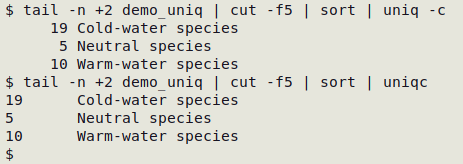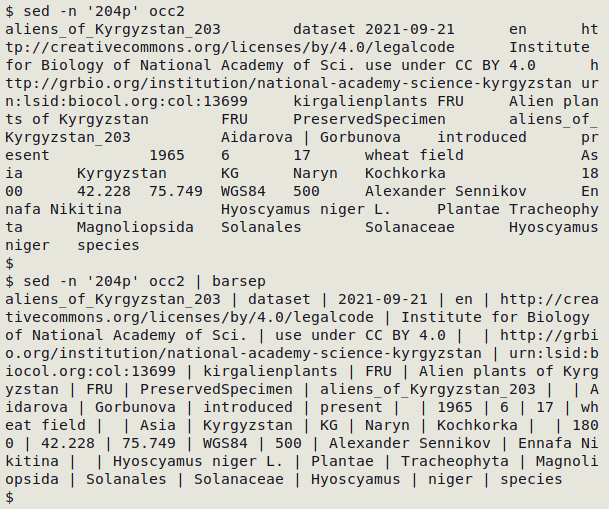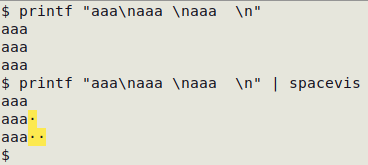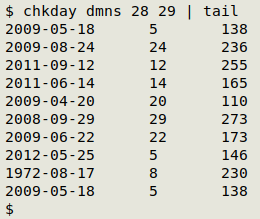On the Extras 1 page:     A data auditing procedure     Reporting results

TSV This marker means that the recipe only works with tab-separated data tables.

Note. This Extras page has recipes for checking "related" fields in a data table. The meaning of "related" will be clear from the examples, below. As a data auditor I've been using these recipes more and more often recently, and I'm not aware of any other simple ways to get the same results.

## Paired fields

The "fldpair" function checks whether paired fields are both filled, neither filled or only one filled in individual records. An example of paired fields is a latitude field and a longitude field. It makes little sense to have a latitude without a longitude or vice-versa, and a data table with these fields should have either both filled or neither filled.

"fldpair" takes 3 arguments: filename, number of first field of pair, number of second field of pair. Field names are taken from the header line of the table. The screenshot below shows "fldpair" at work on 3 pairs of fields in the file "prep".

Tally paired fields    TSV

fldpair() { awk -F"\t" -v one="\$2" -v two="\$3" 'NR==1 {x=\$one; y=\$two; next} (\$one!="") && (\$two!="") {both++} (\$one!="") && (\$two=="") {oneonly++} (\$one=="") && (\$two!="") {twoonly++} (\$one=="") && (\$two=="") {neither++} END {print "neither\t"neither"\n"x" only\t"oneonly"\n"y" only\t"twoonly"\nboth\t"both}' "\$1" | sed 's/\t\$/\t0/' | column -t -s \$'\t'; }Tally triplet fields    TSV

This is the 3-field version of "fldpair", called "fldtriplet":

fldtriplet() {
awk -F"\t" -v one="\$2" -v two="\$3" -v three="\$4" \
'NR==1 {x=\$one; y=\$two; z=\$three; next} \
(\$one != "") && (\$two != "") && (\$three != "") {allthree++} \
(\$one != "") && (\$two == "") && (\$three == "") {oneonly++} \
(\$one == "") && (\$two != "") && (\$three == "") {twoonly++} \
(\$one == "") && (\$two == "") && (\$three != "") {threeonly++} \
(\$one != "") && (\$two != "") && (\$three == "") {onetwoonly++} \
(\$one == "") && (\$two != "") && (\$three != "") {twothreeonly++} \
(\$one != "") && (\$two == "") && (\$three != "") {onethreeonly++} \
(\$one == "") && (\$two == "") && (\$three == "") {none++} \
END {print \
"none\t" none "\n" \
x " only\t" oneonly "\n" \
y " only\t" twoonly "\n" \
z " only\t"threeonly "\n" \
x " + " y " only\t" onetwoonly "\n" \
x " + " z " only\t" onethreeonly "\n" \
y " + " z " only\t" twothreeonly "\nall three\t" allthree}' "\$1" \
| sed 's/\t\$/\t0/' | column -t -s \$'\t'
}## One to many

The "one2many" function is used for paired fields in which each unique, normalised value in the first field should have one and only one value paired with it in the second field. The function is an alternative to tally multiple fields testing, and like "fldpair" takes 3 arguments: filename, number of first field of pair, number of second field of pair. As well as the unique first-field value and multiple second-field values, "one to many" prints the number of records for each case.

Check for one to many in paired fields    TSV

one2many() { awk -F"\t" -v one="\$2" -v many="\$3" '\$one != "" {a[\$one]++; b[\$one][\$many]++} END {for (i in b) {for (j in b[i]) {if (a[i]>b[i][j]) print b[i][j] FS i FS j}}}' "\$1"; }

In the example below, the first field (10) contained locality names in New Zealand and the second field (9) contained their area codes. There should be only one area code for each locality, but "one2many" found 4 records each for 2 different area codes in the file "nz1".This variation on the recipe adds a header with fieldnames to the output, and replaces tabs in the output with [space][bar][space]:

one2manyh() { awk -F"\t" -v one="\$2" -v many="\$3" 'NR==1 {print "No. of records" FS \$one FS \$many; next} \$one != "" {a[\$one]++; b[\$one][\$many]++} END {for (i in b) {for (j in b[i]) {if (a[i]>b[i][j]) print b[i][j] FS i FS j}}}' "\$1" | sed 's/\t/ | /g'; }For one way to fix large numbers of "one2many" errors, see here.

## One (concatenated) to many

This function extends "one2many" so that the first "field" is actually two fields with unique, normalised values, concatenated with a comma. In my data auditing, I often check tables that have a verbal location field, a latitude (or northing) field and a longitude (or easting) field. It sometimes happens that the same location is written in several different ways in the verbal field, like "3 km W of Route 6 and Route 3 junction" and "3km west jcn Routes 6 and 3", but the same way in the coordinate fields. To find these verbal location variants I use "one2manyLL", but the same function can be used for similar, non-geographic fields that are logically related.

Check for one to many in paired fields where the first is two concatenated fields    TSV

one2manyLL() { awk -F"\t" -v lat="\$2" -v lon="\$3" -v verbal="\$4" '\$lat != "" {a[\$lat","\$lon]++; b[\$lat","\$lon][\$verbal]++} END {for (i in b) {for (j in b[i]) {if (a[i]>b[i][j]) print b[i][j] FS i FS j}}}' "\$1"; }

In the table "jch1", easting is field 15, northing is field 16 and location is field 11. The output below only shows the results for the first 4 cases where the same easting/northing combination had different "paired" location strings.As with "one2many", this variation adds a header with fieldnames and replaces tabs in the output with [space][bar][space]:

one2manyLLh() { awk -F"\t" -v lat="\$2" -v lon="\$3" -v verbal="\$4" 'NR==1 {print "No. of records" FS \$lat","\$lon FS \$verbal; next} \$lat != "" {a[\$lat","\$lon]++; b[\$lat","\$lon][\$verbal]++} END {for (i in b) {for (j in b[i]) {if (a[i]>b[i][j]) print b[i][j] FS i FS j}}}' "\$1" | sed 's/\t/ | /g'; }For one way to fix large numbers of "one2manyLL" errors, see here.

## A special date check

The "chkday" function does a very specialised job: in TSV tables with one field containing an ISO 8601 date (YYYY-MM-DD format) and another field containing the corresponding day number for that date (1 to 365, or to 366 in a leap year), "chkday" looks for disagreements between date and day number. The function returns ISO 8601 date, the given day number and the correct day number. More information here.

Check for disagreement between ISO 8601 date and day number    TSV

chkday() { awk -F"\t" -v isodate="\$2" -v dayno="\$3" 'NR>1 && \$isodate != "" && \$dayno != "" {split(\$isodate,a,"-"); b=strftime("%j",mktime(a" "a" "a" 0 0 0")); if (b != sprintf("%03d",\$dayno)) print \$isodate FS \$dayno FS b}' "\$1"; }

In the screenshot below, the table "dmns" has the ISO 8601 date in field 28 and the corresponding day number in field 29, but there are numerous disagreements.